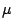A Calculus of Circular Proofs and its Categorical Semantics Luigi Santocanale May 2001

### Abstract:

We present a calculus of proofs, the intended models of which are categories with finite products and coproducts, initial algebras and final coalgebras of functors that are recursively constructible out of these operations, that is,-bicomplete categories. The calculus satisfies the cut elimination and its main characteristic is that the underlying graph of a proof is allowed to contain a certain amount of cycles. To each proof of the calculus we associate a system of equations which has a meaning in every-bicomplete category. We prove that this system admits always a unique solution, and by means of this theorem we define the semantics of the calculus

Available as PostScript, PDF, DVI.